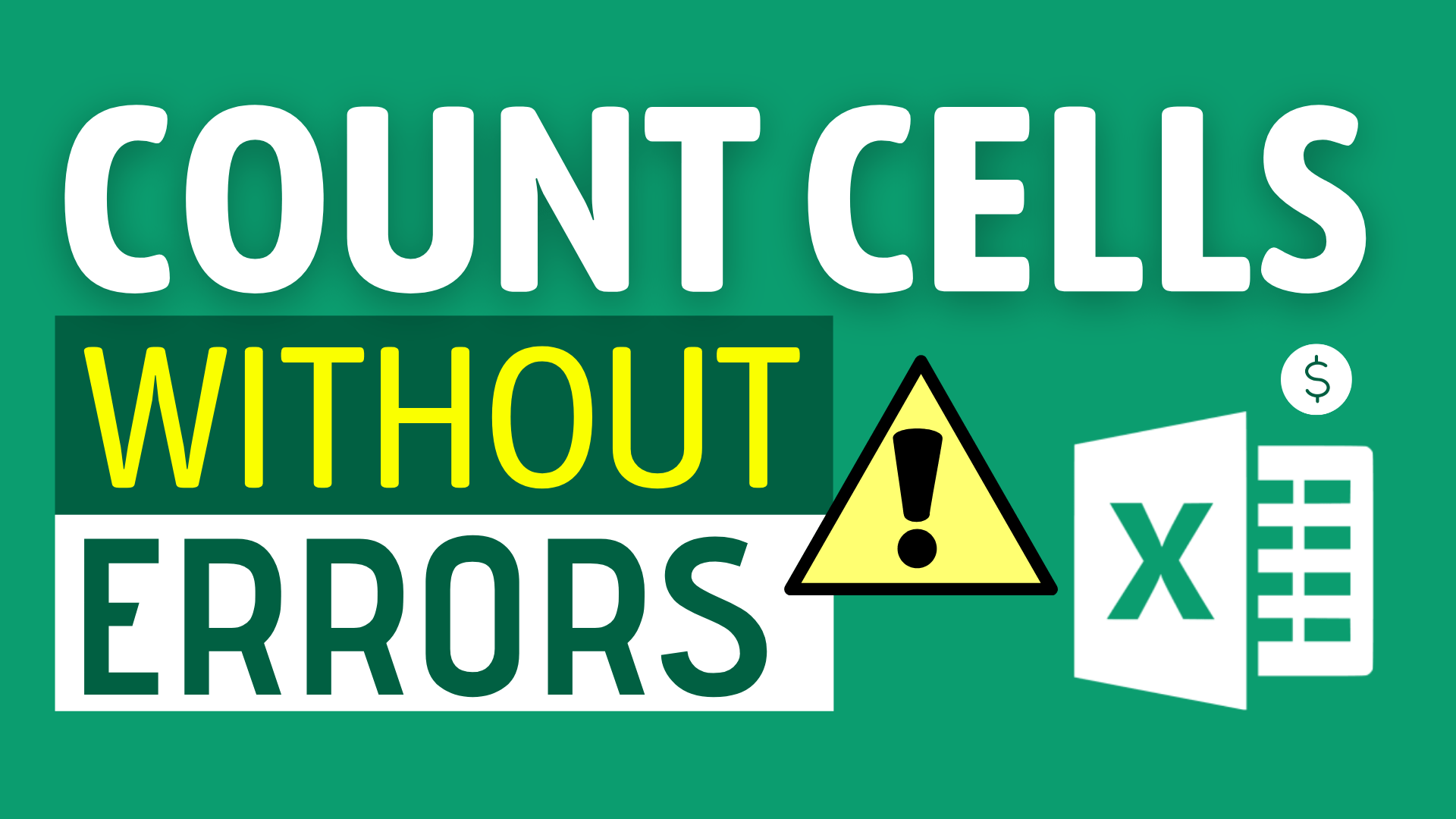# How to Count Cells that do not Contain Errors in Excel## Background

Sometimes you may want to know the number of cells does not contain errors in your workbook.

Indeed this it not difficult at all. You can accomplish it with just one simple formula.

In this article, I will show you how to count number of cells without any error using SUMPRODUCT() and ISERROR().

## Example

What do we want to get?

We would like to find out the number of cells does not contain errors in column B.

As you can see, there are four cells containing errors in column B.

So, we should aim at getting 4 as the result of the formula.

## Expected Outcome

There are two results we can expect from this tutorial:

1. Number of cells does not contain errors excluding N/A errors.
2. Number of cells does not contain errors including N/A errors.

## Formula (ignore N/A errors)

`=SUMPRODUCT(--ISERR(B2:B8))`

#### Logic

Step 1: ISERR will determine if the cell contains error values.

If it does contains error values, it will return TRUE. If it doesn’t, it will return FALSE.

Step 2: SUMPRODUCT will turn the logical value into a numeric value.

If it is “TRUE”, it will turn into 1.

If it is “FALSE”, it will turn into 0.

Step 3: SUMPRODUCT will sum the numeric values.

As you can see from column F, the values of the cell are [1,0,0,1,0,1,0].

So, the sum of them is 1 + 0 + 0 + 1 + 0 + 0 + 1 =3

Step 4: You get the number, which is 3 in this case.

## Formula (include N/A errors)

Are you confused? Do you have the following question?

Are you confused? Do you have the following question?

I saw 4 errors from column B, why is the result only 3?

This is because the ISERR function does not see #N/A as an error. It ignores #N/A when counting errors.

If you would like to include #N/A as an error, then you should go for ISERROR.

`=SUMPRODUCT(--ISERROR(B2:B8))`

The logic is actually 99.9% similar to the former one. The only difference is that it counts #N/A as error.How to Input Time Duration in ExcelHow to Remove Bullets/Numberings in Excel

Popular posts